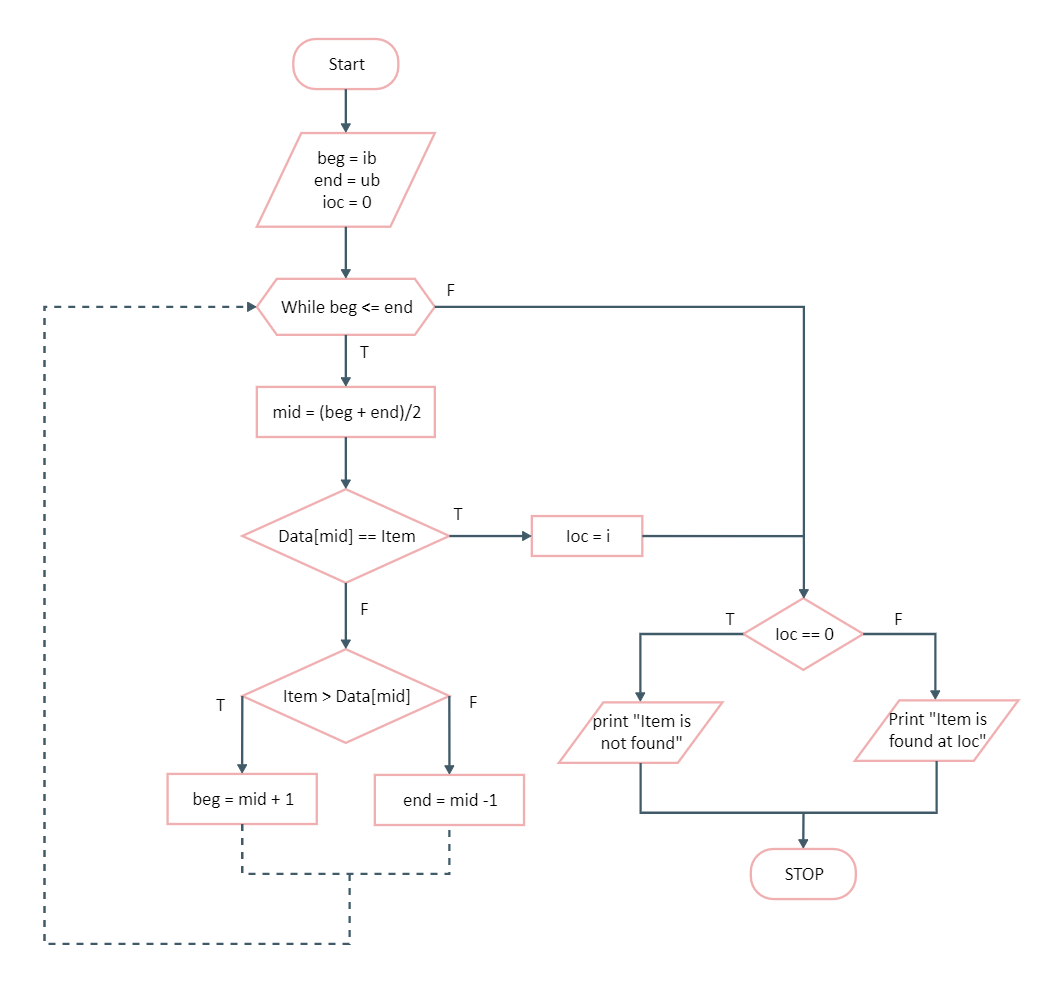Template Community / The Algorithm Flowchart Example

# The Algorithm Flowchart ExampleCharlotte
Published on 2021-07-16
Edit OnlineBinary search follows a divide and conquers approach in which the list is divided into two halves, and the item is compared with the middle element of the list. If the match is found then, the location of the middle element is returned; otherwise, we search into either of the halves depending upon the result produced through the match. The algorithm to understand all this is designed in the image below and can be understood by creating an algorithm where the first step is [INITIALIZE] SET BEG = lower_bound; END = upper_bound, POS = -1. The System will repeat this step while BEG <= END and SET MID = (BEG+END)/2.
Tag
algorithm flowchart
Flowchart Collection
Report
0
93PostRecommended Templates